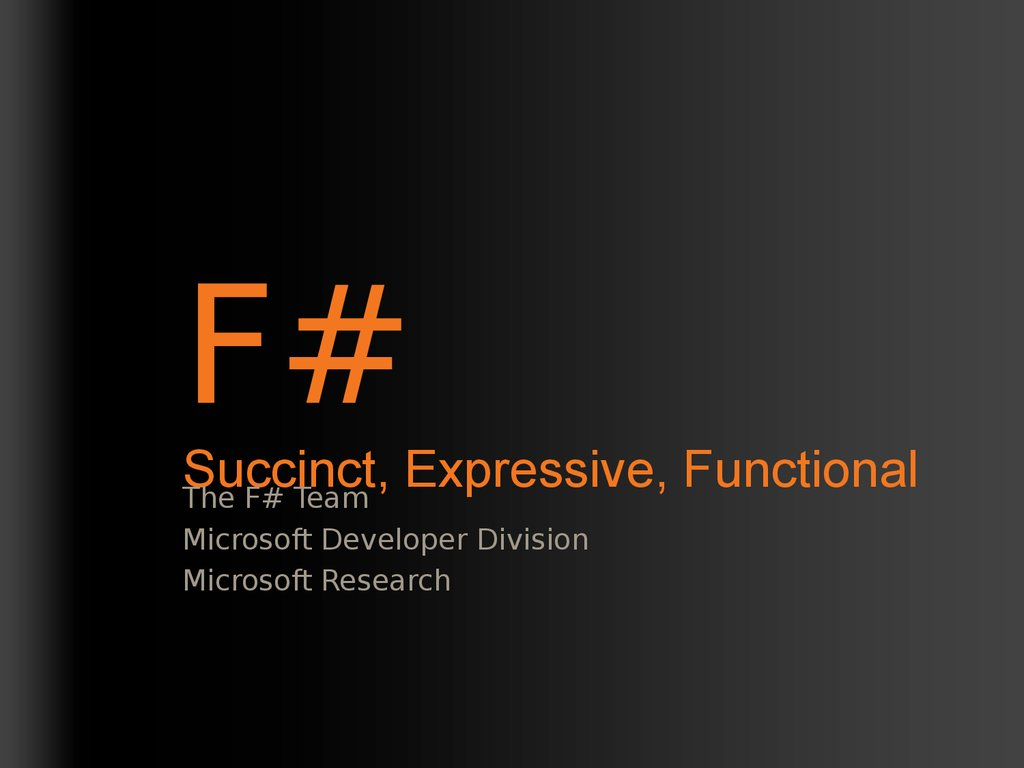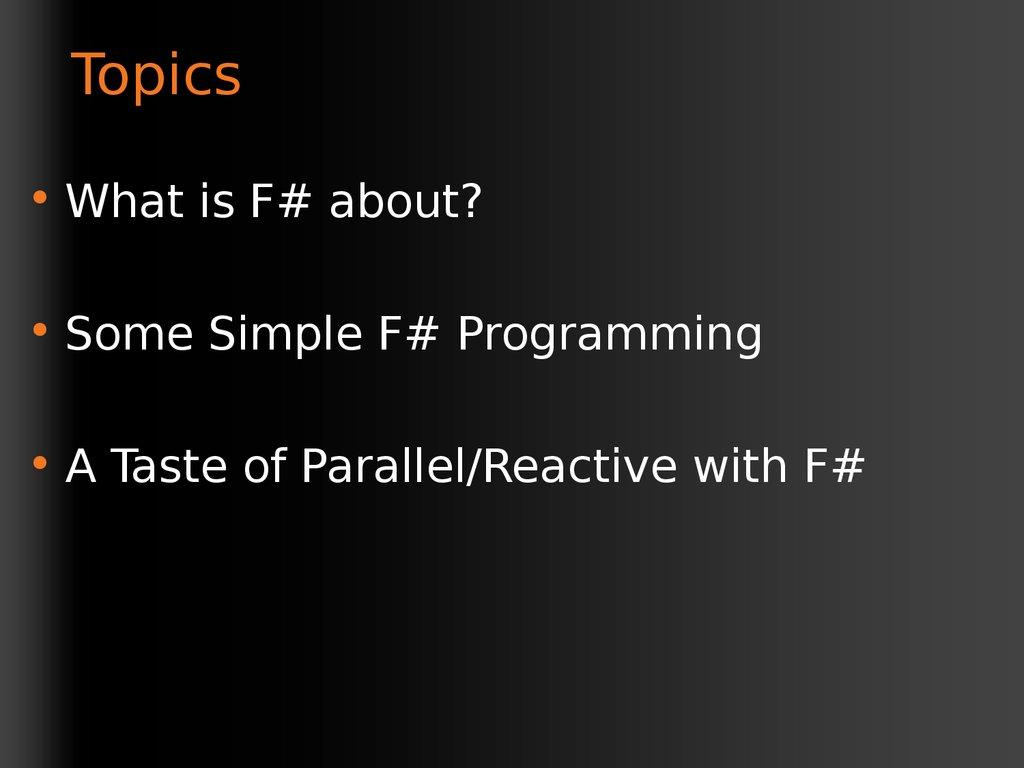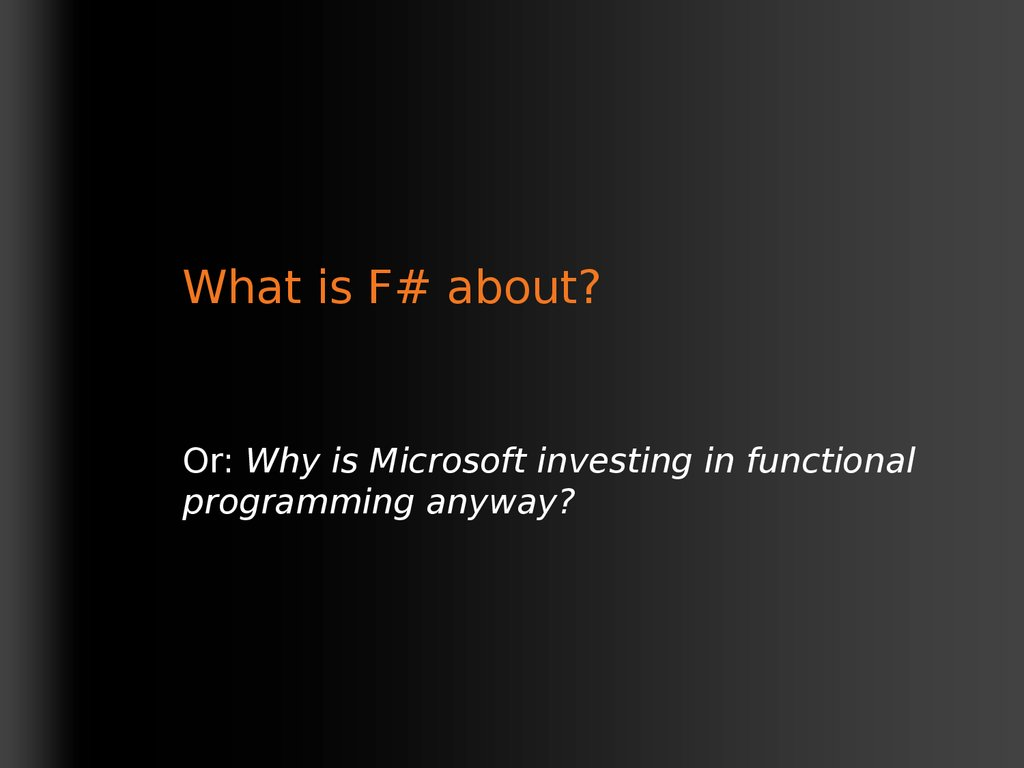# F# Succinct, Expressive, Functional

## 1. F# Succinct, Expressive, Functional

The F# Team
Microsoft Developer Division
Microsoft Research

## 2. Topics

• Some Simple F# Programming
• A Taste of Parallel/Reactive with F#

## 3. What is F# about?

Or: Why is Microsoft investing in functional
programming anyway?

## 8. Code!

//F#
open System
let a = 2
Console.WriteLine a
More Noise
Than Signal!
//C#
using System;
namespace ConsoleApplication1
{
class Program
{
static int a()
{
return 2;
}
static void Main(string[]
args)
{
Console.WriteLine(a);
}
}
}

## 9.

Pleasure
Pain
abstract class Command
{
public virtual void Execute();
}
abstract class MarsRoverCommand : Command
{
let BreakCommand     =
protected MarsRover Rover { get; private set; }

Command(fun rover -> rover.Accelerate(-1.0))
public MarsRoverCommand(MarsRover rover)
{
this.Rover = rover;
let TurnLeftCommand  =
}
}
Command(fun rover -> rover.Rotate(-5.0<degs>))
class BreakCommand : MarsRoverCommand

{
public BreakCommand(MarsRover rover)
: base(rover)
{
}
public override void Execute()
{
Rover.Rotate(-5.0);
}
}
class TurnLeftCommand : MarsRoverCommand
{
public TurnLeftCommand(MarsRover rover)
: base(rover)
{
}
public override void Execute()
{
Rover.Rotate(-5.0);
}
}

type Command = Command of (Rover -> unit)

## 10.

Pleasure
type Expr =
| True
| And of Expr * Expr
| Nand of Expr * Expr
| Or of Expr * Expr
| Xor of Expr * Expr
| Not of Expr
http://stepheneasey.wordpress.com/tag/c/
Pain
public abstract class Expr { }
public abstract class UnaryOp :Expr
{
public Expr First { get; private set; }
public UnaryOp(Expr first)
{
this.First = first;
}
}

public abstract class BinExpr : Expr
{
public Expr First { get; private set; }
public Expr Second { get; private set; }

public BinExpr(Expr first, Expr second)
{
this.First = first;
this.Second = second;
}
}

public class TrueExpr : Expr { }

public class And : BinExpr
{
public And(Expr first, Expr second) : base(first, second) { }
}
public class Nand : BinExpr
{
public Nand(Expr first, Expr second) : base(first, second) { }
}

public class Or : BinExpr
{
public Or(Expr first, Expr second) : base(first, second) { }
}

public class Xor : BinExpr
{
public Xor(Expr first, Expr second) : base(first, second) { }
}

public class Not : UnaryOp
{
public Not(Expr first) : base(first) { }
}

## 11.

Pleasure
Pain
let rotate (x,y,z) = (z,x,y)
Tuple<V,T,U> Rotate(Tuple<T,U,V> t)

return new
Tuple<V,T,U>(t.Item3,t.Item1,t.Item2);
}
let reduce f (x,y,z) =
f x + f y + f z
int Reduce(Func<T,int> f,Tuple<T,T,T> t)

return f(t.Item1) + f(t.Item2) + f
(t.Item3);
}

Love
Programming
In
F#

## 15. F#: Influences

F#
Similar core
language
Similar object
model

I've been coding in F# lately, for a production task.
F# allows you to move smoothly in your
shift slightly towards an object-oriented style, and in
production code, I sometimes have to do some
imperative programming.
project with realistic code. You're never
disappointed in any phase of the project!
Julien Laugel, Chief Software Architect, www.eurostocks.com

## 18. The Path to Mastering F#

Topic
Covered
Today
Scoping and “let”
Tuples
Pattern Matching
Working with Functions
Sequences, Lists, Options
Records and Unions
Basic Imperative Programming
Basic Objects and Types
The F# Libraries
Language Oriented Programming
Parallel and Asynchronous

## 19. Quick Tour

// comment
(* comment *)
/// XML doc comment
let x = 1

## 20. Quick Tour

x +
x x *
x /
x %
-x
y
y
y
y
y
Subtraction
Multiplication
Division
Remainder/modulus
Unary negation
Booleans
not expr Boolean negation
expr && expr
Boolean “and”
expr || expr
Boolean “or”

## 21. Orthogonal & Unified Constructs

Orthogonal & Unified
Constructs
Type inference.
• Let “let” simplify your
The
safety of C# with the
succinctness of a
life… scripting language
Bind a static value
let data = (1,2,3)
Bind a static function
Bind a local value
Bind a local function
let f(a,b,c) =
let sum = a + b + c
let g(x) = sum + x*x
g(a), g(b), g(c)

## 23. Orthogonal & Unified Constructs

Orthogonal & Unified Constructs
• Functions: like delegates + unified and
simple
One simple
Anonymous
mechanism,
predicate = 'a -> bool
(fun x -> x + 1) many
Function value
uses
send = 'a -> unit
let f(x) = x + 1
Declare a
value
= unit -> unit
(f,f)
A pair
of functioncomparer
values = 'a -> 'a -> int
val f : int -> int
hasher = 'a -> int
A function type
equality = 'a -> 'a -> bool

## 24. F# - Functional

let f x = x+1
let pair x = (x,x)
let fst (x,y) = x
let data = (Some [1;2;3], Some [4;5;6])
match data with
| Some(nums1), Some(nums2) -> nums1 @ nums2
| None, Some(nums)
-> nums
| Some(nums), None
-> nums
| None, None
-> failwith "missing!"

## 25. F# - Functional

List.map
Seq.fold
Array.filter
Set.union
Range
Expressions
Lazy.force
Map
LazyList
Events
List via
Async...
query
Array via
query
[ 0..1000 ]
[ for x in 0..10 -> (x, x * x) ]
[| for x in 0..10 -> (x, x * x) |]
IEnumerable
seq { for x in 0..10 -> (x, x * x)
}
via query

Data is
immutable by
default
Values
may not be
changed
Copy &
Not Mutate
Update

## 27. In Praise of Immutability

• Immutable objects can be relied upon
• Immutable objects can transfer between
• Immutable objects can be aliased safely
• Immutable objects lead to (different)
optimization opportunities

## 28. F# - Lists

Generated
Lists
open System.IO
let rec allFiles(dir) =
[ for file in Directory.GetFiles(dir) do
yield file
for sub in Directory.GetDirectories(dir) do
yield! allFiles(sub) ]
allFiles(@"C:\Demo")

## 29. F# - Sequences

On-demand
sequences
open System.IO
let rec allFiles(dir) =
seq
{ for file in Directory.GetFiles(dir) do
yield file
for sub in Directory.GetDirectories(dir) do
yield! allFiles(sub) }
allFiles(@"C:\WINDOWS")
|> Seq.take 100
|> show
Pipelines

## 30. Weakly Typed? Slow?

//F#
#light
open System
let a = 2
Console.WriteLine(a)
//C#
using System;
namespace ConsoleApplication1
{
class Program
{
static int a()
{
return 2;
}
Looks Weakly         static void Main(string[]
args)
typed?
Maybe Dynamic?        {
Console.WriteLine(a);

}
}

F#
Yet rich,
dynamic
Yet succinct

## 32. Objects

Class Types
type ObjectType(args) =
let
let
let
internalValue = expr
internalFunction args = expr
mutable internalState = expr
member x.Prop1 = expr
member x.Meth2 args = expr
Constructing Objects
new FileInfo(@"c:\misc\test.fs")

## 33. F# - Objects + Functional

type Vector2D(dx:double,dy:double)
=
Inputs to
member v.DX = dx
member v.DY = dy
object
constructio
n
Exported
properties
member v.Length = sqrt(dx*dx+dy*dy)
member v.Scale(k) = Vector2D(dx*k,dy*k)
Exported
method

## 34. F# - Objects + Functional

type Vector2D(dx:double,dy:double) =
let norm2 = dx*dx+dy*dy
member v.DX = dx
member v.DY = dy
member v.Length = sqrt(norm2)
member v.Norm2 = norm2
Internal (precomputed)
values and
functions

## 35. F# - Objects + Functional

Immutabl
e inputs
type HuffmanEncoding(freq:seq<char*int>) =
...
< 50 lines of beautiful functional code>
Internal
...
tables
member x.Encode(input: seq<char>) =
Publish
encode(input)
access
member x.Decode(input: seq<char>) =
decode(input)

## 36. F# - Objects + Functional

type Vector2D(dx:double,dy:double) =
let mutable currDX = dx
Internal
state
let mutable currDX = dy
member v.DX = currDX
Publish
internal
state
member v.DY = currDY
member v.Move(x,y) =
currDX <- currDX+x
currDY <- currDY+y
Mutate
internal
state

## 38. The Solution

In parallel programming,
F# is a power tool
• Good Architecture
for good architects and
good developers
• Know your limits (CPU, disk, network, latency)
• Translate Good Architecture into Good
Code with F#
• A great platform
• A massive increase in isolation and immutability
• A massive reduction in mutation

## 39. Async: Simple Examples

Compute 22
and 7 in
parallel
async
async {{ ...
... }}
Async.Parallel
Async.Parallel [WebRequest.Async
[WebRequest.Async
WebRequest.Async
WebRequest.Async
WebRequest.Async
WebRequest.Async
Get these
three web
pages and
wait until all
have come
"http://www.live.com";
"http://www.live.com";
back
"http://www.yahoo.com";
"http://www.yahoo.com";

DEMO

## 41. 8 Ways to Learn

• FSI.exe
http://cs.hubfs.
net
• Samples
Included
Codeplex
Fsharp Samples
• Go to definition
• Lutz’ Reflector
Books
ML

Visit
www.fsharp.net

## 43. Getting F#

• September 2008: CTP released
F# will be a supported language in
Visual Studio 2010
• Next stop: Visual Studio 2010 Beta 1
Look for it soon!

## 44. Questions & Discussion

Questions & Discussion